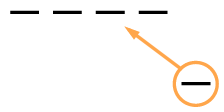### Home > CC2 > Chapter 3 > Lesson 3.3.1 > Problem3-96

3-96.

Represent each operation below by drawing $-$ tiles. Then simplify each expression. Homework Help ✎

1. $- 6 + ( - 2 )$

Gavin had a similar problem. He was told to represent $-4+(-1)$ by drawing $-$ pieces. Look at his diagram below. Then use Gavin's method to solve your problem.1. $- 6 - ( - 2 )$

Gavin was told to represent $-4-(-1)$ by drawing $-$ pieces. Again, look at the diagram below and then use Gavin's method to solve your problem.